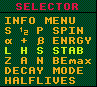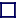### LHS STABILITY MODE INSTRUCTIONS

This plot (and the 'Z A N BEmax' mode plot) plots the decay stabilities as calculated by the differences in atomic masses of neighbouring nuclides, and as such is different to the 'DECAY MODE' plot which displays the OBSERVED decay modes. Any discrepancies between the two are usually as a result of the branching ratio for one decay mode being so low that the decay is un-observed in practice. This applies especially to alpha instabilities where the mass difference is less than 2MeV; here the decays, although theoretically possible, are so improbable as to be unobservable in practice.

If the right hand mouse button is used to select this mode, then (S) just the plain stability plot is plotted with no nuclides highlighted.

If the middle mouse button is used to select this mode then (H) the longest halflife isotope for each atomic number, Z, is enclosed in a green square.

If the left hand mouse button is used to select this mode, then (L) the lightest isobar for each atomic weight is encircled in white.

The lower two options can be plotted together in combination by holding down both left and middle mouse buttons simultaneously.